# Computational implementation of integral equations

Integral equations are solved numerically. One has the Ornstein-Zernike relation,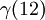$\gamma (12)$ and a closure relation,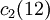$c_2 (12)$ (which incorporates the bridge function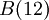$B(12)$). The numerical solution is iterative;

1. trial solution for$\gamma (12)$
2. calculate$c_2 (12)$
3. use the Ornstein-Zernike relation to generate a new$\gamma (12)$ etc.

Note that the value of$c_2 (12)$ is local, i.e. the value of$c_2 (12)$ at a given point is given by the value of$\gamma (12)$ at this point. However, the Ornstein-Zernike relation is non-local. The way to convert the Ornstein-Zernike relation into a local equation is to perform a (fast) Fourier transform (FFT). Note: convergence is poor for liquid densities. (See Ref.s 1 to 6).

## Picard iteration

Picard iteration generates a solution of an initial value problem for an ordinary differential equation (ODE) using fixed-point iteration. Here are the four steps used to solve integral equations:

### Closure relation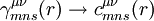$\gamma_{mns}^{\mu \nu} (r) \rightarrow c_{mns}^{\mu \nu} (r)$

(Note: for linear fluids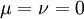$\mu = \nu =0$)

#### Perform the summation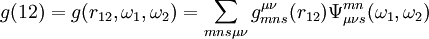$g(12)=g(r_{12},\omega_1,\omega_2)=\sum_{mns\mu \nu} g_{mns}^{\mu \nu}(r_{12}) \Psi_{\mu \nu s}^{mn}(\omega_1,\omega_2)$

where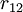$r_{12}$ is the separation between molecular centers and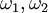$\omega_1,\omega_2$ the sets of Euler angles needed to specify the orientations of the two molecules, with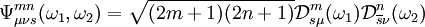$\Psi_{\mu \nu s}^{mn}(\omega_1,\omega_2) = \sqrt{(2m+1)(2n+1)} \mathcal{D}_{s \mu}^m (\omega_1) \mathcal{D}_{\overline{s} \nu}^n (\omega_2)$

with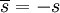$\overline{s} = -s$.

#### Define the variables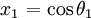$\left. x_1 \right.= \cos \theta_1$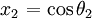$\left. x_2\right.= \cos \theta_2$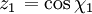$\left. z_1 \right.= \cos \chi_1$$\left. z_2 \right.= \cos \chi_2$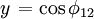$\left. y\right.= \cos \phi_{12}$

Thus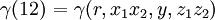$\left. \gamma(12) \right. =\gamma (r,x_1x_2,y,z_1z_2)$.

#### Evaluate

Evaluations of$\gamma (12)$ are performed at the discrete points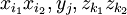$x_{i_1}x_{i_2},y_j,z_{k_1}z_{k_2}$ where the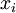$x_i$ are the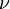$\nu$ roots of the Legendre polynomial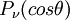$P_\nu(cos \theta)$ where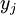$y_j$ are the$\nu$ roots of the Chebyshev polynomial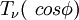$T_{\nu}(\ cos \phi)$ and where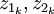$z_{1_k},z_{2_k}$ are the$\nu$ roots of the Chebyshev polynomial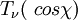$T_{\nu}(\ cos \chi)$ thus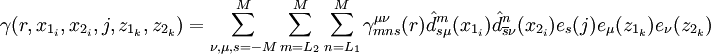$\gamma(r,x_{1_i},x_{2_i},j,z_{1_k},z_{2_k})= \sum_{\nu , \mu , s = -M }^M \sum_{m=L_2}^M \sum_{n=L_1}^M \gamma_{mns}^{\mu \nu} (r) \hat{d}_{s \mu}^m (x_{1_i}) \hat{d}_{\overline{s} \nu}^n (x_{2_i}) e_s(j) e_{\mu} (z_{1_k}) e_{\nu} (z_{2_k})$

where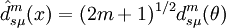$\hat{d}_{s \mu}^m (x) = (2m+1)^{1/2} d_{s \mu}^m(\theta)$

where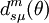$d_{s \mu}^m(\theta)$ is the angular,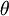$\theta$, part of the rotation matrix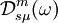$\mathcal{D}_{s \mu}^m (\omega)$, and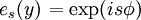$\left. e_s(y) \right.=\exp(is\phi)$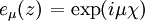$\left. e_{\mu}(z) \right.= \exp(i\mu \chi)$

For the limits in the summations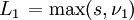$\left. L_1 \right.= \max (s,\nu_1)$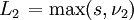$\left. L_2 \right.= \max (s,\nu_2)$

The above equation constitutes a separable five-dimensional transform. To rapidly evaluate this expression it is broken down into five one-dimensional transforms: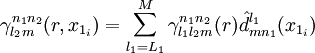$\gamma_{l_2m}^{n_1n_2}(r,x_{1_i})=\sum_{l_1=L_1}^M \gamma_{l_1 l_2 m}^{n_1 n_2}(r) \hat{d}_{m n_1}^{l_1} (x_{1_i})$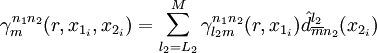$\gamma_{m}^{n_1n_2}(r,x_{1_i},x_{2_i})=\sum_{l_2=L_2}^M \gamma_{l_2 m}^{n_1 n_2}(r,x_{1_i}) \hat{d}_{\overline{m} n_2}^{l_2} (x_{2_i})$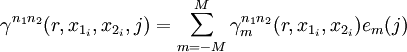$\gamma^{n_1n_2}(r,x_{1_i},x_{2_i},j)=\sum_{m=-M}^M \gamma_{m}^{n_1 n_2}(r,x_{1_i},x_{2_i}) e_m(j)$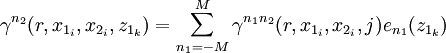$\gamma^{n_2}(r,x_{1_i},x_{2_i},z_{1_k})=\sum_{n_1=-M}^M \gamma^{n_1 n_2}(r,x_{1_i},x_{2_i},j) e_{n_1}(z_{1_k})$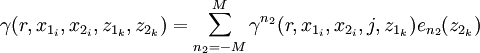$\gamma(r,x_{1_i},x_{2_i},z_{1_k},z_{2_k})=\sum_{n_2=-M}^M \gamma^{n_2}(r,x_{1_i},x_{2_i},j,z_{1_k}) e_{n_2}(z_{2_k})$

Operations involving the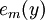$e_m(y)$ and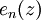$e_n(z)$ basis functions are performed in complex arithmetic. The sum of these operations is asymptotically smaller than the previous expression and thus constitutes a fast separable transform".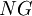$NG$ and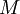$M$ are parameters;$NG$ is the number of nodes in the Gauss integration, and$M$ the the max index in the truncated rotational invariants expansion.

#### Integrate over angles$c_2(12)$

Use Gauss-Legendre quadrature for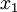$x_1$ and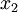$x_2$ Use Gauss-Chebyshev quadrature for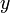$y$,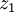$z_1$ and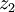$z_2$. Thus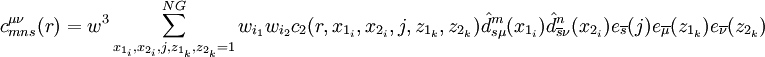$c_{mns}^{\mu \nu} (r) = w^3 \sum_{x_{1_i},x_{2_i},j,z_{1_k},z_{2_k}=1}^{NG} w_{i_1}w_{i_2}c_2(r,x_{1_i},x_{2_i},j,z_{1_k},z_{2_k}) \hat{d}_{s \mu}^m (x_{1_i}) \hat{d}_{\overline{s} \nu}^n (x_{2_i}) e_{\overline{s}}(j) e_{\overline{\mu}} (z_{1_k}) e_{\overline{\nu}} (z_{2_k})$

where the Gauss-Legendre quadrature weights are given by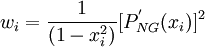$w_i= \frac{1}{(1-x_i^2)}[P_{NG}^{'} (x_i)]^2$

while the Gauss-Chebyshev quadrature has the constant weight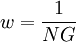$w=\frac{1}{NG}$

### Perform FFT from Real to Fourier space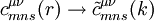$c_{mns}^{\mu \nu} (r) \rightarrow \tilde{c}_{mns}^{\mu \nu} (k)$

This is non-trivial and is undertaken in three steps:

#### Conversion from axial reference frame to spatial reference frame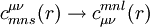$c_{mns}^{\mu \nu} (r) \rightarrow c_{\mu \nu}^{mnl} (r)$

this is done using the Blum transformation (Refs 7, 8 and 9):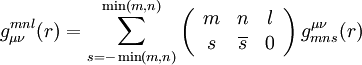$g_{\mu \nu}^{mnl}(r) = \sum_{s=-\min (m,n)}^{\min (m,n)} \left( \begin{array}{ccc} m&n&l\\ s&\overline{s}&0 \end{array} \right)g_{mns}^{\mu \nu} (r)$

#### Fourier-Bessel Transforms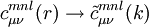$c_{\mu \nu}^{mnl} (r) \rightarrow \tilde{c}_{\mu \nu}^{mnl} (k)$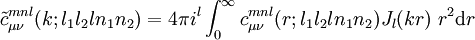$\tilde{c}_{\mu \nu}^{mnl} (k; l_1 l_2 l n_1 n_2) = 4\pi i^l \int_0^{\infty} c_{\mu \nu}^{mnl} (r; l_1 l_2 l n_1 n_2) J_l (kr) ~r^2 {\rm d}r$

(see Blum and Torruella Eq. 5.6 in Ref. 7 or Lado Eq. 39 in Ref. 3), where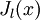$J_l(x)$ is a Bessel function of order$l$. `step-down' operations can be performed by way of sin and cos operations of Fourier transforms, see Eqs. 49a, 49b, 50 of Lado Ref. 3. The Fourier-Bessel transform is also known as a Hankel transform. It is equivalent to a two-dimensional Fourier transform with a radially symmetric integral kernel.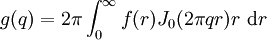$g(q)=2\pi \int_0^\infty f(r) J_0(2 \pi qr)r ~{\rm d}r$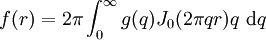$f(r)=2\pi \int_0^\infty g(q) J_0(2 \pi qr)q ~{\rm d}q$

#### Conversion from the spatial reference frame back to the axial reference frame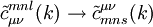$\tilde{c}_{\mu \nu}^{mnl} (k) \rightarrow \tilde{c}_{mns}^{\mu \nu} (k)$

this is done using the Blum transformation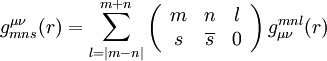$g_{mns}^{\mu \nu} (r) = \sum_{l=|m-n|}^{m+n} \left( \begin{array}{ccc} m&n&l\\ s&\overline{s}&0 \end{array} \right) g_{\mu \nu}^{mnl}(r)$

### Ornstein-Zernike relation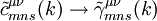$\tilde{c}_{mns}^{\mu \nu} (k) \rightarrow \tilde{\gamma}_{mns}^{\mu \nu} (k)$

For simple fluids: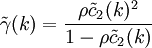$\tilde{\gamma}(k)= \frac{\rho \tilde{c}_2 (k)^2}{1- \rho \tilde{c}_2 (k)}$

For molecular fluids (see Eq. 19 of Lado Ref. 3)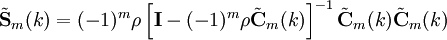$\tilde{{\mathbf S}}_{m}(k) = (-1)^{m}\rho \left[{\mathbf I} - (-1)^{m} \rho \tilde{\mathbf C}_{m}(k) \right]^{-1} \tilde{\mathbf C}_{m}(k)\tilde{\mathbf C}_{m}(k)$

where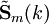$\tilde{{\mathbf S}}_{m}(k)$ and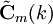$\tilde{\mathbf C}_{m}(k)$ are matrices with elements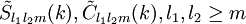$\tilde S_{l_1 l_2 m}(k), \tilde{C}_{l_1 l_2 m}(k), l_1,l_2 \geq m$.

For mixtures of simple fluids (see Ref. 10 Juan Antonio Anta PhD thesis pp. 107--109):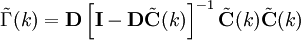$\tilde{\Gamma}(k) = {\mathbf D} \left[{\mathbf I} - {\mathbf D} \tilde{\mathbf C}(k)\right]^{-1} \tilde{\mathbf C}(k)\tilde{\mathbf C}(k)$

### Conversion back from Fourier space to Real space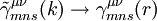$\tilde{\gamma}_{mns}^{\mu \nu} (k) \rightarrow \gamma_{mns}^{\mu \nu} (r)$

(basically the inverse of step 2).

#### Axial reference frame to spatial reference frame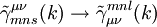$\tilde{\gamma}_{mns}^{\mu \nu} (k) \rightarrow \tilde{\gamma}^{mnl}_{\mu \nu} (k)$

#### Inverse Fourier-Bessel transform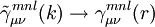$\tilde{\gamma}^{mnl}_{\mu \nu} (k) \rightarrow \gamma^{mnl}_{\mu \nu} (r)$

'Step-up' operations are given by Eq. 53 of Ref. 3. The inverse Hankel transform is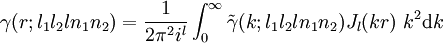$\gamma(r;l_1 l_2 l n_1 n_2)= \frac{1}{2 \pi^2 i^l} \int_0^\infty \tilde{\gamma}(k;l_1 l_2 l n_1 n_2) J_l (kr) ~k^2 {\rm d}k$

#### Change from spatial reference frame back to axial reference frame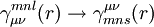$\gamma^{mnl}_{\mu \nu} (r) \rightarrow \gamma_{mns}^{\mu \nu} (r)$.